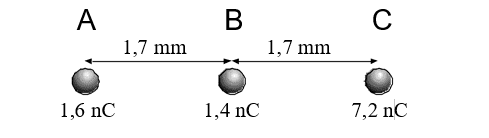# How to calculate net force

• Kofyebs

## Homework Statement

Calculate the net force on A.[/B]## Homework Equations

F=k . (q1 q2)/r^2 , k=9 x 10^9 Nm^2/C^2[/B]

## The Attempt at a Solution

(9*10^9 Nm^2/C^2)(1,4 x 10^-6C)(7,2 x 10^-6C) / 1,7mm^2
= 0,0313.
Could someone tell me what I am missing even if what i did is even correct.
Thanks
[/B]

That’s NOT how you do it!